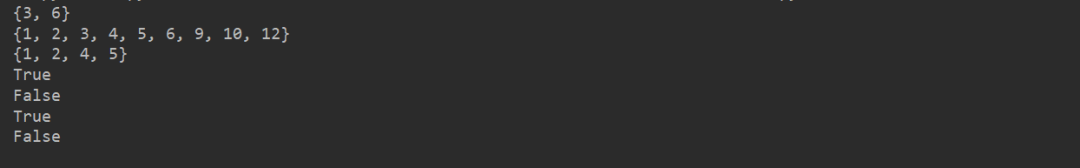# 说说Python集合的那些事儿

## 二、集合常用操作

#### 1. 创建集合

set 的创建可以使用 `{}` 也可以使用 set 函数：

``````s1 = {'a', 'b', 'c', 'a', 'd', 'b'}  # 使用 {}
print(s1)
(['a', 'c', 'b', 'd'])

s2 = set('helloworld')  # 使用 set()，接收一个字符串
print(s2)
set(['e', 'd', 'h', 'l', 'o', 'r', 'w'])
s3 = set(['.mp3', '.mp4', '.rmvb', '.mkv', '.mp3'])  # 使用 set()，接收一个列表
print(s3)
set(['.mp3', '.mkv', '.rmvb', '.mp4'])``````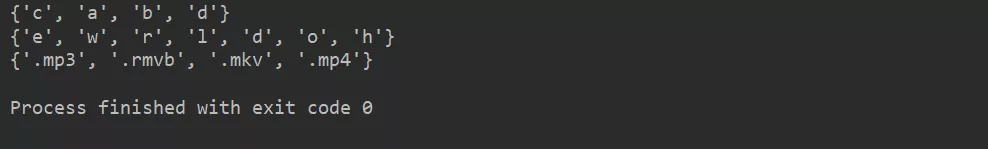#### 2. 遍历集合

``````s = {'a', 'b', 'c', 'a', 'd', 'b'}
for e in s:
print(e)``````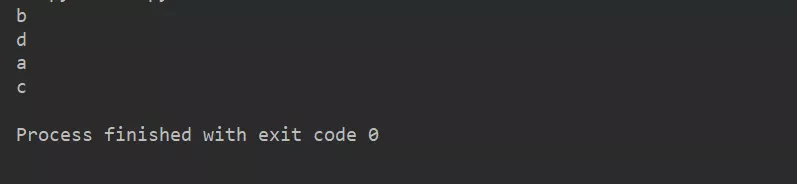#### 3. 添加元素

`add()` 方法可以将元素添加到 set 中，可以重复添加，但没有效果。

``````s = {'a', 'b', 'c', 'a', 'd', 'b'}
print(s)
set(['a', 'c', 'b', 'd'])
print(s)
set(['a', 'c', 'b', 'e', 'd'])
print(s)
set(['a', 'c', 'b', 'e', 'd'])
print(s)
set(['a', 'c', 'b', 4, 'd', 'e'])``````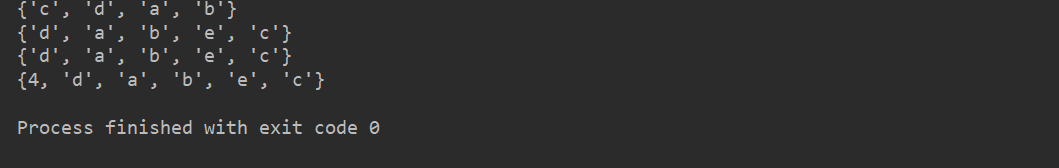#### 4. 删除元素

`remove()` 方法可以删除集合中的元素, 但是删除不存在的元素，会抛出 KeyError，可改用 `discard()`

``````s = {'a', 'b', 'c', 'a', 'd', 'b'}
print(s)
set(['a', 'c', 'b', 'd'])
s.remove('a')  # 删除元素 'a'
print(s)
set(['c', 'b', 'd'])
s.remove('e')  # 删除不存在的元素，会抛出 KeyError``````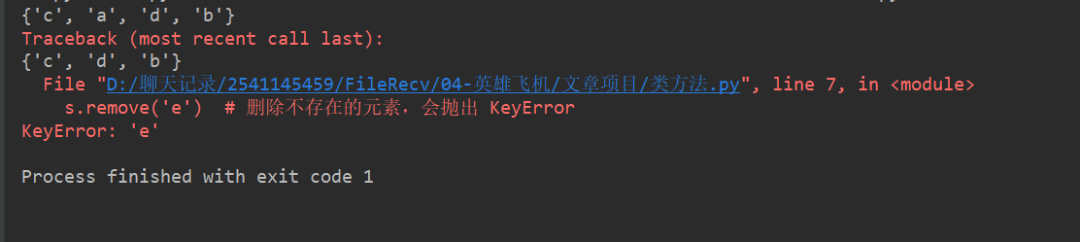``````s = {'a', 'b', 'c', 'a', 'd', 'b'}
print(s)
set(['a', 'c', 'b', 'd'])
s.remove('a')  # 删除元素 'a'
print(s)
set(['c', 'b', 'd'])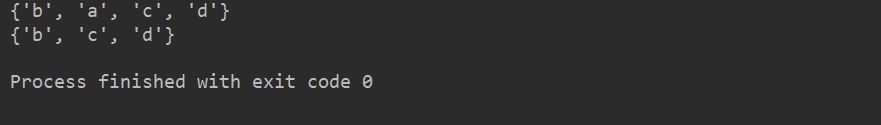## 三、交集/并集/差集

Python 中的集合也可以看成是数学意义上的无序和无重复元素的集合，因此，我们可以对两个集合作交集、并集等。

``````s1 = {1, 2, 3, 4, 5, 6}
s2 = {3, 6, 9, 10, 12}
s3 = {2, 3, 4}
print(s1 & s2 ) # 交集
set([3, 6])
print(s1 | s2)  # 并集
set([1, 2, 3, 4, 5, 6, 9, 10, 12])
print(s1 - s2)  # 差集
set([1, 2, 4, 5])
print(s3.issubset(s1))  # s3 是否是 s1 的子集

print(s3.issubset(s2))  # s3 是否是 s2 的子集

print(s1.issuperset(s3))  # s1 是否是 s3 的超集

print(s1.issuperset(s2))  # s1 是否是 s2 的超集``````